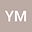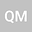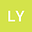Asymptotic behavior of the Boussinesq equation with nonlocal weak damping and arbitrary growth nonlinear function
•••Yichun Mo
Northwest Normal University
Author ProfileLijuan YAO
Northwest Normal University
Author Profile## Abstract

In this paper, we consider the asymptotic behavior of the Boussinesq equation with nonlocal weak damping when the nonlinear function is arbitrary polynomial growth. We firstly prove the well-posedness of solution by means of the monotone operator theory. At the same time, we obtain the dissipative property of the dynamical system (E ,S( t)) associated with the problem in the space H 0 2 ( Ω ) × L 2 ( Ω ) and D ( A 3 4 ) × H 0 1 ( Ω ) , respectively. After that, the asymptotic smoothness of the dynamical system (E ,S( t)) and the further quasi-stability are demonstrated by the energy reconstruction method. Finally, different from  we show not only existence of the finite global attractor but also existence of the generalized exponential attractor.